# 图像处理 避免光照的影响

## 去光照不均匀matlab 算法和代码放出

2017-12-08 23:03:01 baolinq 阅读数 16583
• ###### 目标跟踪

学会自己查阅opencv doxygen文档 学会编写opencv代码实现简单图像处理变换 初步了解图像算法及机器视觉基础 运用计算机视觉相关知识和opencv库来构建简单的应用程序。

231人学习 金圣韬
免费试看

主要看第三部分就可以，前面是一些其他常见 的基本图像方法。基于二维伽马函数的光照不均匀图像自适应校正算法1.直方图均衡化2.retenix去雾算法

Retinex理论在彩色图像增强、图像去雾、去光照不均匀影响、彩色图像恢复方面拥有很好的效果。网上有很多博文讲这个算法，是个非常经典的算法，试验测试结果截图：Retinex效果图3. 基于二维伽马函数的光照不均匀图像自适应校正算法

（1）  利用多尺度高斯函数去除场景的光照分量

（2）  然后构造了一种二维伽马函数，并利用光照分量的分布特性调整二维伽马函数的参数，降低光照过强区域的亮度值，提高光照过暗区域图像的亮度值，最终实现对光照不均匀图像的自适应校正处理。γ的指数应该是m-I(x,y)，所以说论文里的公式还是要自己去推导一下~~Rgb2hsv,对h分量多尺度高斯卷积（高斯滤波），二维gamma函数校正，hsv2rgb，结束。

clc,close all;
tic;
figure;
imshow(im);
title('原图');
[h,s,v]=rgb2hsv(im);    %转到hsv空间，对亮度h处理
% 高斯滤波
HSIZE= min(size(im,1),size(im,2));%高斯卷积核尺寸
q=sqrt(2);
SIGMA1=15;%论文里面的c
SIGMA2=80;
SIGMA3=250;
F1 = fspecial('gaussian',HSIZE,SIGMA1/q);
F2 = fspecial('gaussian',HSIZE,SIGMA2/q) ;
F3 = fspecial('gaussian',HSIZE,SIGMA3/q) ;
gaus1= imfilter(v, F1, 'replicate');
gaus2= imfilter(v, F2, 'replicate');
gaus3= imfilter(v, F3, 'replicate');
gaus=(gaus1+gaus2+gaus3)/3;    %多尺度高斯卷积，加权，权重为1/3
% gaus=(gaus*255);
figure;
imshow(gaus,[]);
title('光照分量');
%二维伽马卷积
m=mean(gaus(:));
[w,height]=size(v);
out=zeros(size(v));
gama=power(0.5,((m-gaus)/m));%根据公式gamma校正处理，论文公式有误
out=(power(v,gama));
figure;
imshow(out,[]);
rgb=hsv2rgb(h,s,out);   %转回rgb空间显示
figure;
imshow(rgb);
title('处理结果')
toc;

注意：刘志成, 王殿伟, 刘颖,等. 基于二维伽马函数的光照不均匀图像自适应校正算法[J]. 北京理工大学学报, 2016, 36(2):191-196.

## 图像处理常用算法(基础)

2018-08-12 23:15:22 echo_1994 阅读数 31283
• ###### 目标跟踪

学会自己查阅opencv doxygen文档 学会编写opencv代码实现简单图像处理变换 初步了解图像算法及机器视觉基础 运用计算机视觉相关知识和opencv库来构建简单的应用程序。

231人学习 金圣韬
免费试看

在实际的图像分割中，往往只用到一阶和二阶导数，虽然，原理上，可以用更高阶的导数，但是，因为噪声的影响，在纯粹二阶的导数操作中就会出现对噪声的敏感现象，三阶以上的导数信息往往失去了应用价值。二阶导数还可以说明灰度突变的类型。在有些情况下，如灰度变化均匀的图像，只利用一阶导数可能找不到边界，此时二阶导数就能提供很有用的信息。二阶导数对噪声也比较敏感，解决的方法是先对图像进行平滑滤波，消除部分噪声，再进行边缘检测。不过，利用二阶导数信息的算法是基于过零检测的，因此得到的边缘点数比较少，有利于后继的处理和识别工作。

各种算子的存在就是对这种导数分割原理进行的实例化计算，是为了在计算过程中直接使用的一种计算单位。

1.Sobel算子

其主要用于边缘检测，在技术上它是以离散型的差分算子，用来运算图像亮度函数的梯度的近似值， Sobel算子是典型的基于一阶导数的边缘检测算子，由于该算子中引入了类似局部平均的运算，因此对噪声具有平滑作用，能很好的消除噪声的影响。Sobel算子对于象素的位置的影响做了加权，与Prewitt算子、Roberts算子相比因此效果更好。

Sobel算子包含两组3x3的矩阵，分别为横向及纵向模板，将之与图像作平面卷积，即可分别得出横向及纵向的亮度差分近似值。实际使用中，常用如下两个模板来检测图像边缘。

2. Isotropic Sobel算子

Sobel算子另一种形式是(Isotropic Sobel)算子，加权平均算子，权值反比于邻点与中心点的距离，当沿不同方向检测边缘时梯度幅度一致，就是通常所说的各向同性Sobel(Isotropic Sobel)算子。模板也有两个，一个是检测水平边沿的 ，另一个是检测垂直平边沿的 。各向同性Sobel算子和普通Sobel算子相比，它的位置加权系数更为准确，在检测不同方向的边沿时梯度的幅度一致。

3. Roberts算子

Roberts边缘算子是一个2x2的模板，采用的是对角方向相邻的两个像素之差。从图像处理的实际效果来看，边缘定位较准，对噪声敏感。适用于边缘明显且噪声较少的图像分割。Roberts边缘检测算子是一种利用局部差分算子寻找边缘的算子,Robert算子图像处理后结果边缘不是很平滑。经分析，由于Robert算子通常会在图像边缘附近的区域内产生较宽的响应，故采用上述算子检测的边缘图像常需做细化处理，边缘定位的精度不是很高。

4. Prewitt算子

Prewitt算子是一种一阶微分算子的边缘检测，利用像素点上下、左右邻点的灰度差，在边缘处达到极值检测边缘，去掉部分伪边缘，对噪声具有平滑作用 。其原理是在图像空间利用两个方向模板与图像进行邻域卷积来完成的，这两个方向模板一个检测水平边缘，一个检测垂直边缘。

对数字图像f(x，y)，Prewitt算子的定义如下：

G(i)=|[f(i-1,j-1)+f(i-1,j)+f(i-1，j+1)]-[f(i+1,j-1)+f(i+1，j)+f(i+1，j+1)]|

G(j)=|[f(i-1,j+1)+f(i,j+1)+f(i+1，j+1)]-[f(i-1,j-1)+f(i,j-1)+f(i+1，j-1)]|

Prewitt算子对噪声有抑制作用，抑制噪声的原理是通过像素平均，但是像素平均相当于对图像的低通滤波，所以Prewitt算子对边缘的定位不如Roberts算子。

5.Laplacian算子

Laplace算子是一种各向同性算子，二阶微分算子，在只关心边缘的位置而不考虑其周围的象素灰度差值时比较合适。Laplace算子对孤立象素的响应要比对边缘或线的响应要更强烈，因此只适用于无噪声图象。存在噪声情况下，使用Laplacian算子检测边缘之前需要先进行低通滤波。所以，通常的分割算法都是把Laplacian算子和平滑算子结合起来生成一个新的模板。

拉式算子用来改善因扩散效应的模糊特别有效，因为它符合降制模型。扩散效应是成像过程中经常发生的现象。

Laplacian算子一般不以其原始形式用于边缘检测，因为其作为一个二阶导数，Laplacian算子对噪声具有无法接受的敏感性；同时其幅值产生算边缘，这是复杂的分割不希望有的结果；最后Laplacian算子不能检测边缘的方向；所以Laplacian在分割中所起的作用包括：（1）利用它的零交叉性质进行边缘定位；（2）确定一个像素是在一条边缘暗的一面还是亮的一面；一般使用的是高斯型拉普拉斯算子（Laplacian of a Gaussian,LoG)，由于二阶导数是线性运算，利用LoG卷积一幅图像与首先使用高斯型平滑函数卷积改图像，然后计算所得结果的拉普拉斯是一样的。所以在LoG公式中使用高斯函数的目的就是对图像进行平滑处理，使用Laplacian算子的目的是提供一幅用零交叉确定边缘位置的图像；图像的平滑处理减少了噪声的影响并且它的主要作用还是抵消由Laplacian算子的二阶导数引起的逐渐增加的噪声影响。

6.Canny算子

该算子功能比前面几种都要好，但是它实现起来较为麻烦，Canny算子是一个具有滤波，增强，检测的多阶段的优化算子，在进行处理前，Canny算子先利用高斯平滑滤波器来平滑图像以除去噪声，Canny分割算法采用一阶偏导的有限差分来计算梯度幅值和方向，在处理过程中，Canny算子还将经过一个非极大值抑制的过程，最后Canny算子还采用两个阈值来连接边缘。

Canny边缘检测算法

step1: 用高斯滤波器平滑图象；

step2: 用一阶偏导的有限差分来计算梯度的幅值和方向；

step3: 对梯度幅值进行非极大值抑制

step4: 用双阈值算法检测和连接边缘

(1)图象边缘检测必须满足两个条件：一能有效地抑制噪声；二必须尽量精确确定边缘的位置。

(2)根据对信噪比与定位乘积进行测度，得到最优化逼近算子。这就是Canny边缘检测算子。

(3)类似与Marr（LoG）边缘检测方法，也属于先平滑后求导数的方法。

## 《数字图像处理》复习提纲

2019-06-08 12:59:38 weixin_37545024 阅读数 785
• ###### 目标跟踪

学会自己查阅opencv doxygen文档 学会编写opencv代码实现简单图像处理变换 初步了解图像算法及机器视觉基础 运用计算机视觉相关知识和opencv库来构建简单的应用程序。

231人学习 金圣韬
免费试看

1、数字图像与图像处理

2、数字图像处理的特点：

1、数字图像处理的目的

2、数字图像处理的主要内容

## 第2章 数字图像处理基础

1、采样

2、量化

3、采样与量化参数的选择

4、图像数字化设备

### 2.2 色度学基础与颜色模型

1、色度学基础

2、颜色模型

（1）RGB模型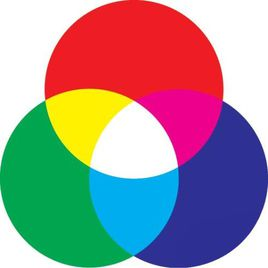（2）CIE XYZ模型
F=x•X+y•Y+z•Z, x=X/(X+Y+Z), y=Y/(X+Y+Z), z=Z/(X+Y+Z)
（3）Lab颜色模型

（4）HSI颜色模型

（5）CMY与CMYK颜色模型
（C，M，Y）=（1，1，1）-（R，G，B）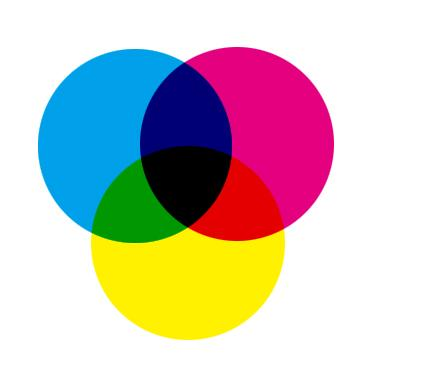（6）YUV电视信号彩色坐标系统
Y表示亮度信号，U、V表示色差
（7）YCbCr模型

3、颜色变换

1、位图

2、位图有关术语

### 2.4 图像文件格式

BMP图像文件格式：
（1）四部分组成：位图文件头、位图信息头、调色板、图像数据
（2）字节对齐，4byte整数倍
（3）上下倒置存放

### 2.5 OpenCV编程简介

OpenCV = Open Source Computer Vision Library
1、OpenCV简介 不考
2、OpenCV的安装与配置 不考
3、OpenCV的数据结构 不太可能考

4、读入、显示和存储图像

#include <iostream>
#include <opencv2/core/core.hpp>
#include <opencv2/highgui/highgui.hpp>
using namespace std;
using namespace cv;
int main(){
Mat image, result;
cout << "size: " << image.size().height() << " , " << image.size().width << endl;
if(!image.data){
cout << "read image file failed!" << endl;
}
namedWindow("Original Image");
imshow("Original Image", image);
flip(image, result,1);
namedWindow("Output Image");
imshow("Output Image", result);
imwrite("output.bmp", result);
waitKey(0);
return 0
}


5、操作图像像素
（1）at方法

void colorReduce(Mat& inputImage, Mat& outputImage, int div){
outputImage = inputImage.clone();
int rows = outputImage.rows;
int cols = outputImage.cols;
for(int i=0;i<rows;i++){
for(int j=0;j<cols;j++){
outputImage.at<Vec3b>(i,j) = outputImage.at<Vec3b>(i,j)/div*div+div/2;
outputImage.at<Vec3b>(i,j) = outputImage.at<Vec3b>(i,j)/div*div+div/2;
outputImage.at<Vec3b>(i,j) = outputImage.at<Vec3b>(i,j)/div*div+div/2;
}
}
}


（2）使用迭代器

void colorReduce(Mat& inputImage, Mat& outputImage, int div){
outputImage = inputImage.clone();
Mat_<Vec3b>::iterator it = inputImage.begin<Vec3b>();
Mat_<Vec3b>::iterator it = inputImage.end<Vec3b>();
Mat_<Vec3b> cimage = outputImage;
Mat_<Vec3b>::iterator itout = cimage.begin();
Mat_<Vec3b>::iterator itoutend = cimage.end();
for(; it != itend; it++,itout++){
(*itout)=(*it)/div*div+div/2;
(*itout)=(*it)/div*div+div/2;
(*itout)=(*it)/div*div+div/2;
}
}


（3）使用指针

void colorReduce(Mat& inputImage, Mat& outputImage, int div){
outputImage = inputImage.clone();
int rows = outputImage.rows;
int cols = outputImage.cols;
if(outputImage.isContinuous()){
cols = cols * rows;
rows = 1;
}
for(int i=0;i<rows;i++){
uchar * dataout = outputImage.ptr<uchar>(i);
for(int j=0;j<cols;j++){
dataout[j] = dataout[j]/div*div+div/2;
}
}
}


4、整行整列像素值的赋值

img.row(i).setTo(Scalar(255));
img.col(i).setTo(Scalar(255));


6、图形交互和媒体接口HighGui 不太可能考

## 第3章 图像增强

### 3.1 灰度变换

1、灰度线性变换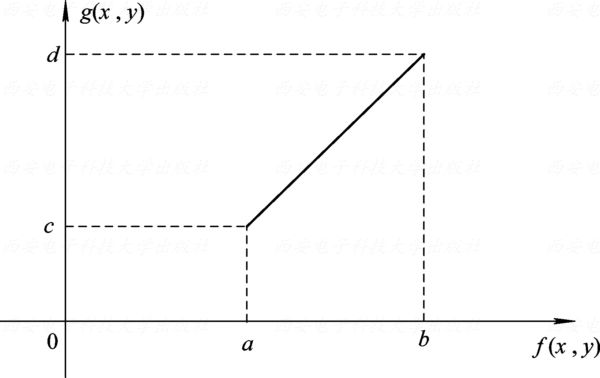2、分段线性变换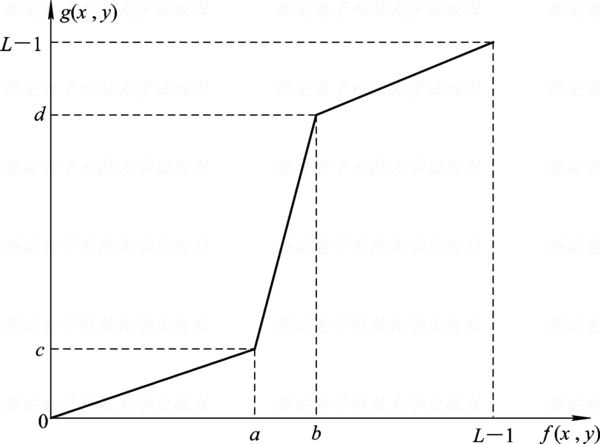3、非线性变换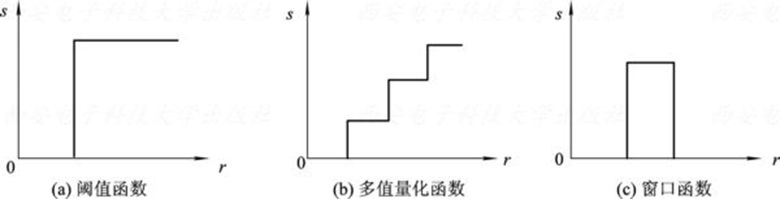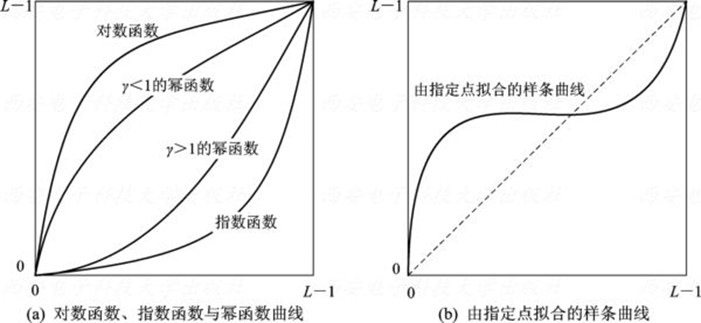### 3.2 直方图修正

1、直方图的基本概念

2、直方图的性质
（1）丢失位置信息，保留统计信息
（2）图像与灰度直方图是一对多的关系
（3）灰度直方图具有可加性
3、直方图的计算
（1）初始化：数组零值初始化
（2）统计：将量化灰度值映射为数组下标，遍历图像将对应数组元素的值++
（3）归一化：数组所有元素除以像素总数

4、直方图均衡化
（1）基本思想：把原始图像的直方图变换为均匀分布的形式，从而增加图像灰度的动态范围，以达到增强图像对比度的效果。经过均衡化处理的图像，其灰度级出现的概率相同，此时图像的熵最大，图像包含的信息量最大。
（2）推导
$s=T(r)$
s和r分别为灰度变换前后的灰度级，$T(r)$单调递增且有界[0,1]
$η=T(ξ)$，则有
$F_{η}(s) = P(η<s) = P(ξ<r) = \int_{-\infty}^{r}P_{r}(x)dx$

$p_{s}(s)=[p_{r}(r)•\frac{\mathrm{d} r}{\mathrm{d} s}]_{r=T^{-1}(s)}$

$p_s(s)=1$

$\mathrm{d} s=p_r(r)\mathrm{d}r$

$T(r)=s=\int_0^rp_r(ω)\mathrm{d}ω$

（3）数字图像的直方图均衡化

k rk nk pr=T(rk) sk=int[(L-1)sk+0.5] s rk->sk ps(sk)
0 0 790 0.19 0.19 0.19→1 0→1 -
1 1 1023 0.25 0.44 0.144→3 1→3 0.19
2 2 850 0.21 0.65 0.65→5 2→5 -
3 3 656 0.16 0.81 0.81→6 3→6 0.25
4 4 329 0.08 0.89 0.89→6 4→6 -
5 5 245 0.06 0.95 0.95→7 5→7 0.21
6 6 122 0.03 0.98 0.98→7 6→7 0.24
7 7 81 0.02 1 1→7 7→7 0.11

5、直方图规定化

rk,zl pr(rk) pz(zl) sk=T(rk) vl=G(zl) 单映射rk→zl 单映射ps(sk) 组映射rk→zl 组映射ps(sk)
0 0.19 - 0.19 - r0→r3 0 r0→r3 0
1 0.25 - 0.44 - r1→r3 0 r1→r5 0
2 0.21 - 0.65 - r2→r5 0 r2→r5 0
3 0.16 0.2 0.81 0.2 r3→r5 0.44 r3→r7 0.19
4 0.08 - 0.89 - r4→r7 0 r4→r7 0
5 0.06 0.5 0.95 0.7 r5→r7 0.37 r5→r7 0.46
6 0.03 - 0.98 - r6→r7 0 r6→r7 0
7 0.02 0.3 1 1 r7→r7 0.19 r7→r7 0.35

### 3.3 图像平滑

1、图像噪声
（1）噪声分类

（2）特点

2、模板卷积

3、邻域平均
Box模板：
$\frac{1}{9}\begin{bmatrix} 1 & 1 & 1 \\ 1 & 1* & 1 \\ 1 & 1 & 1 \end{bmatrix}$

$\frac{1}{16}\begin{bmatrix} 1 & 2 & 1 \\ 2 & 4* & 2 \\ 1 & 2 & 1 \end{bmatrix}$

4、中值滤波

（1）不影响阶跃信号、斜坡信号、连续个数少于窗口长度一半的脉冲受到抑制，三角函数顶部变平。
（2）中值滤波的输出与输入噪声的密度分布有关
（3）中值滤波频谱特性起伏不大，可以认为中值滤波之后，信号频谱基本不变

5、图像平均

### 3.4 图像锐化

1、微分法

（1）梯度算子

$\mathrm{▽}\mathbf{f}(x,y)=\begin{bmatrix}\frac{\partial f}{\partial x} & \frac{\partial f}{\partial y}\end{bmatrix}^T$

$\mathrm{▽} f(x,y)=|\mathrm{▽}\mathbf{f}(x,y)|=(G_x^2+G_y^2)^{\frac 1 2}$

$φ(x,y)=arctan(\frac {G_y} {G_x})$

$\mathrm{▽} f(x,y) \approx |f(x+1,y)-f(x,y)|+|f(x,y+1)-f(x,y)|$
Robert交叉算子
$\mathrm{▽} f(x,y) \approx |f(x+1,y+1)-f(x,y)|+|f(x,y+1)-f(x+1,y)|$

$\left \{\begin{matrix} G_x=\frac{f(x+1,y)-f(x-1,y)}{2} \\ \\ G_y=\frac{f(x,y+1)-f(x,y-1)}{2} \end{matrix}\right.$

Roberts $\begin{bmatrix}0* & -1 \\ 1 & 0\end{bmatrix}$ $\begin{bmatrix}-1* & 0 \\ 0 & 1\end{bmatrix}$ 各向同性；对噪声敏感；模板尺寸为偶数，中心位置不明显
Prewitt $\begin{bmatrix}-1 & 0 & 1 \\ -1 & 0* & 1 \\ -1 & 0 & 1 \end{bmatrix}$ $\begin{bmatrix}-1 & -1 & -1 \\ 0 & 0* & 0 \\ 1 & 1 & 1\end{bmatrix}$ 引入了平均因素，对噪声有抑制作用；操作简便
Sobel $\begin{bmatrix}-1 & 0 & 1 \\ -2 & 0* & 2 \\ -1 & 0 & 1 \end{bmatrix}$ $\begin{bmatrix}-1 & -2 & -1 \\ 0 & 0* & 0 \\ 1 & 2 & 1\end{bmatrix}$ 引入了平均因素，增强了最近像素的影响，噪声抑制效果比Prewitt要好
Krisch $\begin{bmatrix}-3 & -3 & 5 \\ -3 & 0* & 5 \\ -3 & -3 & 5 \end{bmatrix}$ $\begin{bmatrix}-3 & -3 & -3 \\ -3 & 0* & -3 \\ 5 & 5 & 5\end{bmatrix}$ 噪声抑制效果要好；需求出8个方向的响应（这里只给出两个方向的模板）
Isotropic Sobel $\begin{bmatrix}-1 & 0 & 1 \\ -\sqrt 2 & 0* & \sqrt 2 \\ -1 & 0 & 1 \end{bmatrix}$ $\begin{bmatrix}-1 & -\sqrt 2 & -1 \\ 0 & 0* & 0 \\ 1 & \sqrt 2 & 1\end{bmatrix}$ 权值反比于邻点与中心点的距离，检沿不同方向边缘时梯度幅度一致，即具有各向同性

（2）拉普拉斯算子

$\Delta f=\nabla ^2f=\frac{\partial ^2f}{\partial ^2x}+\frac{\partial ^2f}{\partial ^2y}$

$\nabla ^2f \approx f(x+1,y)+f(x-1,y)+f(x,y+1)+f(x,y-1)-4f(x,y)$

$\begin{bmatrix} 0 & -1 & 0 \\ -1 & 4* & -1 \\ 0 & -1 & 0 \end{bmatrix} \begin{bmatrix} -1 & -1 & -1 \\ -1 & 8* & -1 \\ -1 & -1 & -1 \end{bmatrix} \begin{bmatrix} 1 & -2 & 1 \\ -2 & 4* & -2 \\ 1 & -2 & 1 \end{bmatrix}$

（1）微分模板的权系数之和为0，使得灰度平坦区的响应为0。平滑模板的权系数都为正，和为1，这使得灰度平坦区的输出与输入相同。
（2）一阶微分模板在对比度较大的点产生较高的响应，二阶微分模板在对比度大的点产生零交叉。一阶微分一般产生更粗的边缘，二阶微分则产生更细的边缘。相对一阶微分而言，二阶微分对细线、孤立的点等小细节有更强的响应。
（3）平滑模板的平滑或去噪程度与模板的大小成正比，跳变边缘的模糊程度与模板的大小成正比。
2、非锐化滤波

$g(x,y)=f(x,y)-f_s(x,y)$

3、高频增强滤波

$f_{hb}(x,y)=Af(x,y)+Kg(x,y)$
$f_{hb}(x,y)$表示输出图像，$g(x,y)$$f(x,y)$的一个高通图像，A>=0，0<=K<=1。K在0.2~0.7之间取值时，高频增强滤波的效果最好；A足够大时，图像锐化作用削弱，输出图像接近原始图像整数倍；特别地，当A=1，K=1时为常用的拉普拉斯高频增强模板。

$\begin{bmatrix} 0 & -1 & 0 \\ -1 & 5* & -1 \\ 0 & -1 & 0 \end{bmatrix} \begin{bmatrix} -1 & -1 & -1 \\ -1 & 9* & -1 \\ -1 & -1 & -1 \end{bmatrix} \begin{bmatrix} 1 & -2 & 1 \\ -2 & 5* & -2 \\ 1 & -2 & 1 \end{bmatrix}$

### 3.5 伪彩色处理

1、灰度分层法：类似于等高线、卫星云图、天气预报图等
2、灰度变换法：红外热成像仪等
3、频域滤波法：在频率域进行伪彩色处理

### 3.6 同态滤波

$f(x,y)=i(x,y)r(x,y)$

$\mathrm{ln}f(x,y)=\mathrm{ln}i(x,y)+\mathrm{ln}r(x,y)$

$F_f(u,v)=F_i(u,v)+F_r(u,v)$

$H(u,v)=(\gamma _H-\gamma _L)[1- e^{-c\frac{D^2(u,v)}{D_0^2}}]+\gamma _L$
$\gamma _H$$\gamma _L$用于控制滤波强度，c用于控制滤波器斜面的锐化程度，$D^2(u,v)$为频谱上点（u，v)到频谱中心的距离，$D_0^2$为截止半径。

$g(x,y)=exp\{F^{-1}[F_f(u,v)H(u,v)]\}$

## 第4章 图像的几何变换

### 4.1 几何变换基础

1、概述
2、齐次坐标

3、二维图像几何变换矩阵
$P=TP_0 \\ P_0=\begin{bmatrix}x_1 & x_2 & ... & x_n \\ y_1 & y_2 & ... & y_n \\ 1 & 1 & ... & 1\end{bmatrix} \\ \\ P_=\begin{bmatrix}Hx_1' & Hx_2' & ... & Hx_n' \\ Hy_1' & Hy_2' & ... & Hy_n' \\ H & H & ... & H\end{bmatrix} \\ T=\begin{bmatrix}a & b & p \\ c & d & q \\ 0 & 0 & 1\end{bmatrix}$

### 4.2 图像比例缩放

1、图像比例放缩变换

（1）图像的比例缩小
$I(x,y)=F(int(cx),int(cy)), c=\frac 1 k$
（2）图像的非比例缩小
$I(x,y)=F(int(c_1x),int(c_2y)), c_1=\frac 1 {k_1}, c_2=\frac 1 {k_2}$
（3）图像的比例放大

$g(x,y)=(1-q)[(1-p)f([x],[y]+1)+pf([x]+1,[y])]+q[(1-p)f([x],[y]+1)+pf([x]+1,[y]+1)]$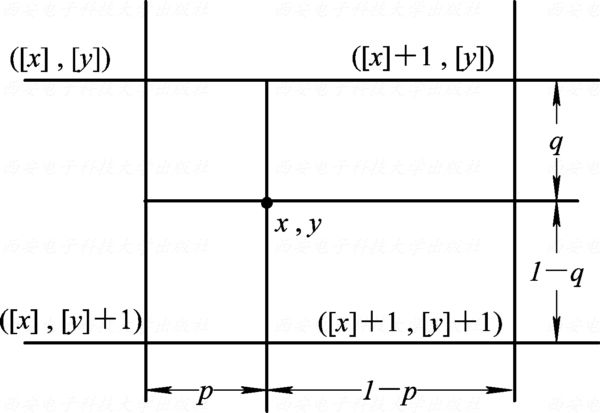2、比例缩放的实现 （略）

1、图像的旋转变换

2、图像旋转的实现（略）

### 4.6 图像复合变换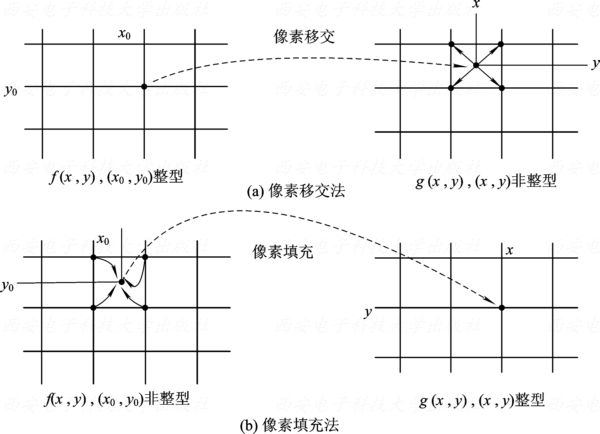1、透视变换

2、其他变换

（1）非矩形像素坐标的转换

（2）图像错切

（3）图像卷绕

### 4.8 几何畸变的矫正（1）利用控制点对图像数据建立一个模拟几何畸变的数学模型，以建立畸变图像空间与标准空间的对应关系
（2）用这种对应关系把畸变空间中的全部元素变换到标准空间中去。

（1）直接成图法

$(x,y)=\boldsymbol f(u,v)$
（2）间接成图法（重采样成图法）

$(u,v)=\boldsymbol f^{-1}(x,y)$

$\left \{ \begin{matrix} u=g_u(x,y)=\sum_{i=0}^{n}\sum_{j=0}^{n-i}a_{ij}x^iy^j \\ \\ v=g_v(x,y)=\sum_{i=0}^{n}\sum_{j=0}^{n-i}b_{ij}x^iy^j \end{matrix} \right.$

$\left \{ \begin{matrix} \varepsilon_u=\sum_{k=1}^K(u_k-\sum_{i=0}^{n}\sum_{j=0}^{n-i}a_{ij}x_k^iy_k^j)^2 \\ \\ \varepsilon_v=\sum_{k=1}^K(v_k-\sum_{i=0}^{n}\sum_{j=0}^{n-i}b_{ij}x_k^iy_k^j)^2 \end{matrix} \right.$

## 第5章 频域处理

### 5.1 频域与频域变换

$f(x)\begin{matrix}正变换 \\ \Leftrightarrow \\ 逆变换\end{matrix}A(\omega),\Phi(\omega) \rightarrow F(\omega)$
5.2 傅里叶变换
1、连续函数的傅里叶变换

2、离散傅里叶变换

$\mathscr{F}[f(x)]=F(u)=\sum_{x=0}^{N-1}f(x)e^{-j\frac {2\pi ux}{N}} \\ \mathscr{F}^{-1}[F(u)]=f(x)=\frac 1 N \sum_{u=0}^{N-1}F(u)e^{j\frac {2\pi ux}{N}} \\ \mathscr{F}[f(x)]=F(u)=\sum_{x=0}^{N-1}f(x)[cos(\frac {2\pi} Nux)-j\cdot sin(\frac {2\pi} Nux)] \\ \mathscr{F}^{-1}[F(u)]=f(x)=\frac 1 N \sum_{u=0}^{N-1}F(u)[cos(\frac {2\pi} Nux)+j\cdot sin(\frac {2\pi} Nux)] \\$

$\mathscr{F}[f(x,y)]=F(u,v)=\sum_{x=0}^{M-1}\sum_{y=0}^{N-1}f(x,y)e^{-j2\pi (\frac {ux} M+\frac {vy} N)} \\ \mathscr{F}^{-1}[f(x,y)]=F(u,v)=\frac 1 {MN}\sum_{u=0}^{M-1}\sum_{v=0}^{N-1}F(u,v)e^{j2\pi (\frac {ux} M+\frac {vy} N)}$
3、离散傅里叶变换的性质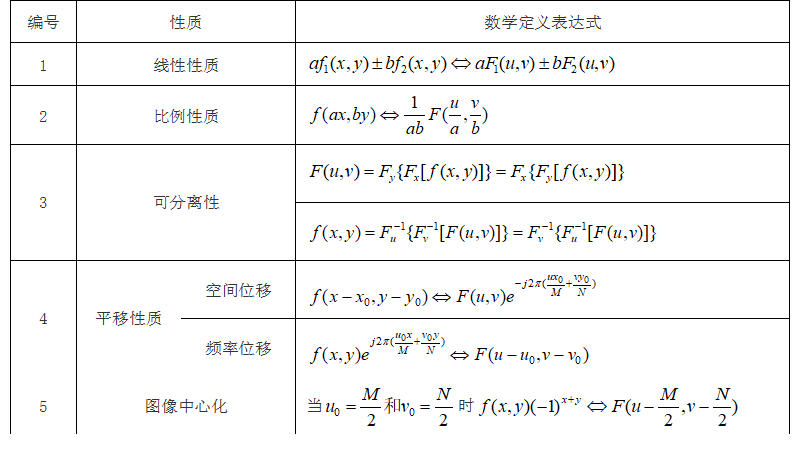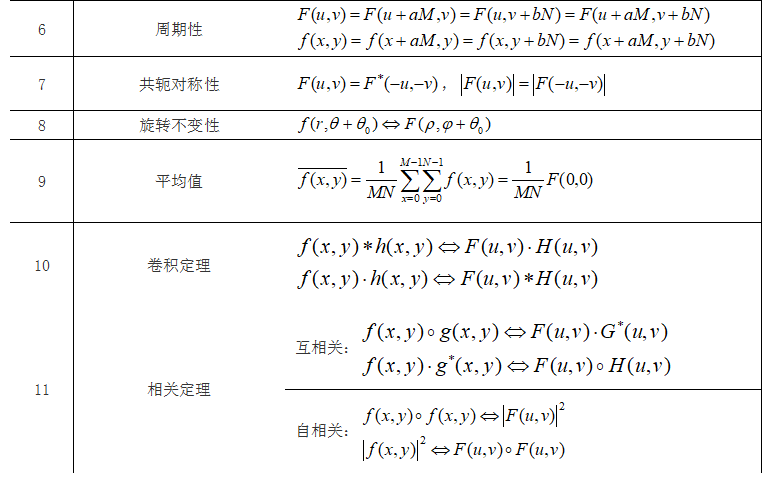4、离散傅里叶变换的openCV 不考

### 5.3 频域变换的一般表达式

1、可分离变换

$F(u,v)=\sum_{x=0}^{M-1}\sum_{y=0}^{N-1}f(x,y)\cdot g(x,y,u,v) \\ f(x,y)=\sum_{u=0}^{M-1}\sum_{v=0}^{N-1}F(u,v)\cdot h(x,y,u,v)$

$g(x,y,u,v)=g_1(x,u)\cdot g_2(y,v) \\ h(x,y,u,v)=h_1(x,u)\cdot h_2(y,v)$

2、图像变换的矩阵表示
$\boldsymbol {F=PfQ} \\ \boldsymbol {f=P^{-1}FQ^{-1}} \\ P(u,x)=g_1(x,u)=e^{-j\frac {2\pi ux} M} \\ Q(y,v)=g_2(y,v)=e^{-j\frac {2\pi vy} N}$

### 5.4 离散余弦变换（DCT）

1、一维离散余弦变换
DCT变换核定义：
$\begin{matrix} g(x,u)=C(u)\sqrt {\frac 2 N cos \frac {(2x+1)u\pi} {2N}} \\ \\ C(u)=\left \{ \begin{matrix} \frac 1 {\sqrt 2}, u=0 \\ \\ 1,else\end{matrix}\right. \end{matrix}$

$F(u)=\sum_{x=0}^{N-1}f(x)g(x,u) \\ \boldsymbol {F=Gf}(矩阵形式，G(u,x)=g(x,u)）$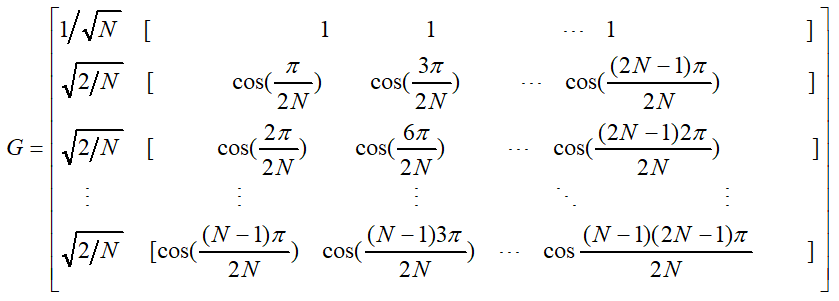DCT逆变换IDCT：
$h(x,u)=g(x,u)=C(u)\sqrt {\frac 2 N cos \frac {(2x+1)u\pi} {2N}} \\ f(x)=\sum_{u=0}^{N-1}F(u)h(x,u)$
2、二维离散余弦变换
2D-DCT：
$g_1(x,u)= \sqrt {\frac 2 M} C(u) cos \frac {(2x+1)u\pi} {2M} \\ g_2(y,v)= \sqrt {\frac 2 N} C(v) cos \frac {(2x+1)v\pi} {2N} \\ g(x,y,u,v)=g_1(x,u)g_2(y,v) \\ F(u,v)=\sum_{x=0}^{M-1}\sum_{y=0}^{N-1}f(x,y)g(x,y,u,v)$
2D-IDCT：
$h_1(x,u)=g_1(x,u) \\ h_2(y,v)=g_2(y,v) \\ h(x,y,u,v)=h_1(x,u)\cdot h_2(y,v) \\ f(x,y)\sum_{u=0}^{M-1}\sum_{v=0}^{N-1}F(u,v)h(x,y,u,v)$

### 5.5 频域中图像处理的实现

1、理解数字图像的频谱图

2、频域图像处理步骤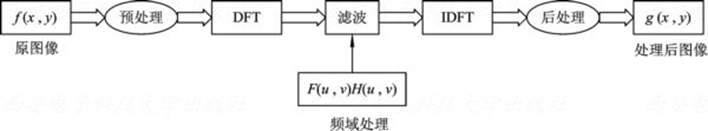3、频域滤波

## 第6章 数学形态学处理

1、数学形态学

2、基本符号和术语

### 6.2 二值形态学

1、腐蚀
$X\ominus S=\{x\in X |S+x\subseteq X\}$

2、膨胀
$X\oplus S=\bigcup\{X+s|s\in S\}=\bigcup\{S+x|x\in X\}=\{x|(S^v+x) \cap X\neq \varnothing\}$

$X\ominus(Y\cap Z)=(X\ominus Y)\cap(X\ominus Z) \\ X\oplus(Y\cap Z)=(X\oplus Y)\cap(X\oplus Z) \\ (X\cap Y)\ominus Z\supset(X\ominus Z)\cap (Y\ominus Z) \\ (X\cap Y)\oplus Z=(X\oplus Z)\cap (Y\oplus Z) \\ (X\cup Y)\ominus Z=(X\ominus Z)\cup (Y\ominus Z) \\ (X\cup Y)\oplus Z\subseteq(X\oplus Z)\cup (Y\oplus Z)$
3、开闭运算

（1）对偶性：$(X^C○S)^C=X\cdot S; (X^C\cdot S)=X○S$
（2）扩展性：$X○S\subseteq X \subseteq X\cdot S$

（3）单调性：$X\subseteq Y \Rightarrow X\cdot S\subseteq Y\cdot S, X○ S\subseteq Y○ S$
$Y\subseteq Z\wedge Z\cdot Y=Z \Rightarrow X\cdot Y\subseteq X \cdot Z$
（4）平移不变性：$(X+h)\cdot S=(X\cdot S)+h$
$(X+h)○ S=(X○ S)+h$
$X\cdot (S+h)=X\cdot S$
$X○ (S+h)=X○ S$
（5）等幂性：$(X\cdot S)\cdot S = X\cdot S,(X○ S)○ S = X○ S$
（6）开闭运算与集合的关系：
$(\bigcup_{i=0}^{n}X_i)○S\supseteq \bigcup_{i=0}^{n}(X_i○S)$
$(\bigcap_{i=0}^{n}X_i)○S\subseteq \bigcap_{i=0}^{n}(X_i○S)$
$(\bigcup_{i=0}^{n}X_i)\cdot S\supseteq \bigcup_{i=0}^{n}(X_i\cdot S)$
$(\bigcap_{i=0}^{n}X_i)\cdot S\subseteq \bigcap_{i=0}^{n}(X_i\cdot S)$
4、击中击不中变换
$X\odot S=\{x|S_1+x\subseteq X \wedge S_2+x\subseteq X^C\}=(X\ominus S_1)\cap(X^C\ominus S_2)$

### 6.3 灰值形态学

1、灰值腐蚀
$(f\ominus b)(s,t)=min\{f(s+x,t+y)-b(x,y)|s+x,t+y\in D_f \wedge x,y \in D_b\}$

2、灰值膨胀
$(f\oplus b)(s,t)=max\{f(s-x,t-y)+b(x,y)|s-x,t-y\in D_f \wedge x,y \in D_b\}$

$(f\oplus b)^C=f^C \ominus b^V$
$(f\ominus b)^C=f^C \oplus b^V$
3、灰值开闭运算

### 6.4 形态学的应用

1、形态学滤波

2、骨架提取

（1）基于腐蚀和开运算的细化

$S_k(X)=\bigcup\{S_k(X)|k=0,1,...,n\}$

$S_k(X)=(X\ominus kB)-[(X\ominus kB)○ B] \\ 递推公式：X\ominus kB=(X\ominus (k-1)B)\ominus B$

$S(X)=\bigcup\{(X\ominus kB)-[(X\ominus kB)○B]|k=0,1,2,...,n\}$

（2）快速形态学细化算法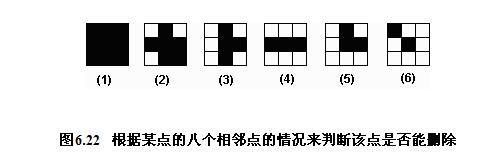（1）（2）（4）（6）都不能删除，（2）（4）可以删除

## 第7章 图像分割

### 7.1 阈值分割

1、概述

（1）种类：全局阈值、局部阈值、动态阈值（自适应阈值）
（2）阈值分割的要素：$T=T[x,y,f(x,y),p(x,y)]$, f(x,y)表示灰度值，p(x,y)表示某种局部性质。
2、全局阈值

（1）极小点阈值法：取直方图谷底位置作为阈值
（2）迭代阈值法：初始阈值$T_1$→像素划分为$G_1$$G_2$→计算平均灰度$\mu_1$$\mu_2$→计算新的阈值$T_2=\frac {\mu_1+\mu_2} 2$→迭代→终止条件$|T_2-T_1| <T_0$
（3）最优阈值法：选择一个阈值使得总的分类误差概率降到最低。
$p(z)$为灰度概率密度函数，$p_1(z)$$p_2(z)$分别为背景和目标灰度条件概率密度函数，$P_1$$P_2$为背景和目标像素出现的概率，那么由全概率公式得：
$p(z)=P_1p_1(z)+P_2p_2(z)$

$E_1(T)=\int_{-\infty}^Tp_1(z)\mathrm{d}z$

$E_2(T)=\int_T^{+\infty}p_2(z)\mathrm{d}z$

$E(T)=P_2E_1(T)+P_1E_2(T)$

$T=\frac {\mu_1+\mu_2} 2+\frac {\sigma^2} {\mu_1-\mu_2}ln(\frac{P_2}{P_1})$
（4）Otsu方法：使阈值分割后各个像素类的类间方差最大。

（5）p参数法

3、局部阈值
（1）直方图变换法

（2）散射图

4、动态阈值

1、区域生长

2、区域分裂与合并

### 7.3 边缘检测

1、微分算子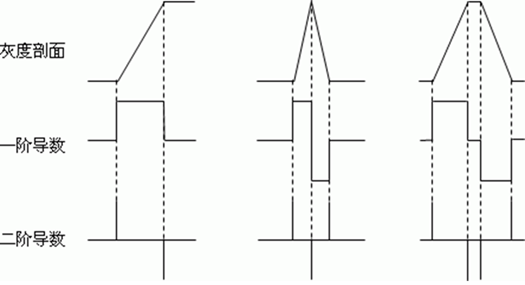（1）梯度算子：
Krisch、Prewitt，均可沿8个方向
（2）高斯-拉普拉斯算子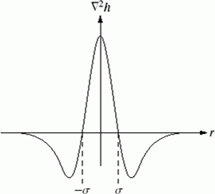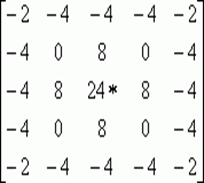（3）Canny边缘检测
Canny算子是一个非常有效的边界检测算子。
①首先对灰度图像进行高斯平滑。
②接着计算每个像素的梯度幅值和梯度方向。
③ 然后细化边缘。如果当前像素的梯度幅值不高于梯度方向上两个邻点的梯度幅值，则抑制该像素响应，这种方法称之为非最大抑制。
④ Canny算子使用两个幅值阈值，高阈值用于检测梯度幅值大的强边缘，低阈值用于检测梯度幅值较小的弱边缘。低阈值通常取为高阈值的一半。
⑤ 边缘细化后，就开始跟踪具有高幅值的轮廓。
⑥ 最后，从满足高阈值的边缘像素开始，顺序跟踪连续的轮廓段，把与强边缘相连的弱边缘连接起来。
2、边界连接

3、哈夫变换

（1）直线检测
y=ax+b→b=-ax+y→$\left \{\begin{matrix}b=-ax_i+y_i \\ b=-ax_j+y_j\end{matrix}\right.$（2）圆的检测：
$\left \{ \begin{matrix} a=x-rcos\theta \\ b=y-rsin\theta \end{matrix} \right.$
（3）任意曲线的检测：类似做法

1、区域标记

2、轮廓提取

3、轮廓追踪

### 7.5 分水岭分割

1、基本分水岭算法

2、Vincent-Soille算法

### 7.7 图像分割实例

（1）高斯滤波、中值滤波或开闭运算消噪
（2）消除非均匀光照：背景照度估计、灰值形态学、同态滤波
（2）区域分割

## 第8章 图像特征与理解

，全局特征用于描述图像或目标的颜色或形状等整体特征，局部特征指一些能够稳定出现并具有良好可区分性的特征。

### 8.1 图像的基本特征

1、几何特征
（1）位置和方向

（2）周长

（3）面积

①已知区域的行程编码，只需把值为1的行程长度相加，即为区域面积。
②若给定边界的某种表示，则相应连通区域的面积应为区域外边界包围的面积，减去它的内边界包围的面积（空的面积）
$y_k=y_0+\sum_{i=0}^k\Delta y_i \\ \Delta y_i=\left \{\begin{matrix}-1,\varepsilon _i = 1,2,3 \\ 0,\varepsilon _i = 0,4\\ 1,\varepsilon _i = 5,6,7 \\\end{matrix}\right. \\ \Delta x_i=\left \{\begin{matrix}-1,\varepsilon _i = 0,1,7 \\ 0,\varepsilon _i = 2,6\\ 1,\varepsilon _i = 3,4,5 \\\end{matrix}\right. \\ A=\sum_{i=1}^n(y_{i-1}\Delta x_i+a)$

（4）长轴和短轴

（5）距离

2、形状特征
（1）矩形度=物体面积/最小外接矩形面积

（2）圆形度：

3、球状性=内切圆半径/外接圆半径
4、偏心率=区域长轴/区域短轴
5、形状描述子
（1）边界链码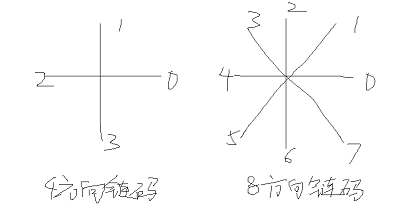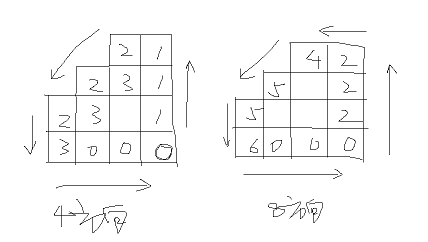（2）一阶差分链码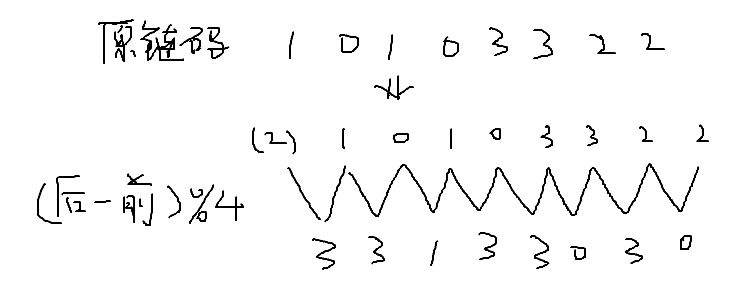（3）傅里叶描述子

### 8.2 角点特征

1、Moravec算法

$E(u,v)=\sum_{(x,y)\in W}(I(x+u,y+v)-I(x,y))^2$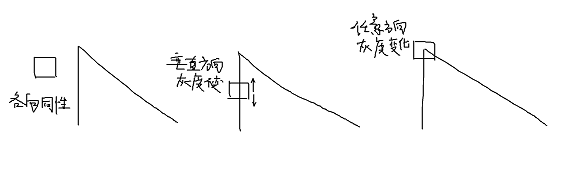2、Harris算法

$E(u,v)=\sum_{(x,y)\in W}w(x,y)(I(x+u,y+v)-I(x,y))^2 \\ w(x,y)=e^{-\frac {(x-x_0)^2+(y-y_0)^2} {2\sigma^2}} \\ 对I(x+u,y+v)进行泰勒展开：I(x+u,y+v)=I(x,y)+uI_x+vI_y \\ 代入上面的式子得:E(u,v)=[u,v]\boldsymbol M[u,v]^T \\ \boldsymbol M=\sum_{(x,y)\in W}w(x,y)\begin{bmatrix}I_x^2 & I_xI_y \\ I_xI_y & I_y^2 \end{bmatrix}=\begin{bmatrix}a & b \\ c & d \end{bmatrix}$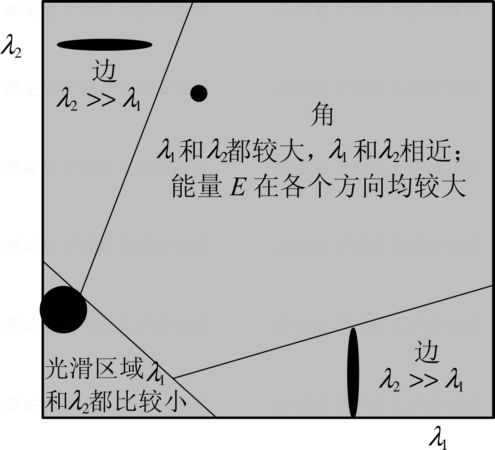3、SUSAN算法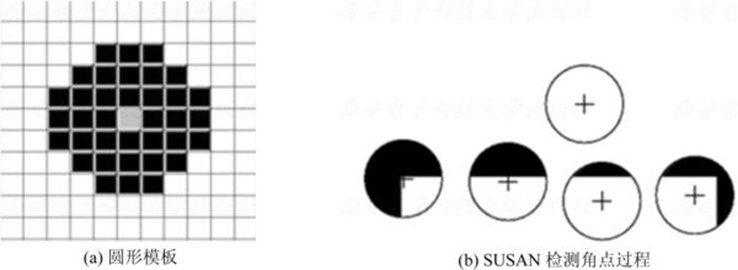## 第9章图像编码

### 9.1 图像编码概述

1、图像编码的基本原理和方法
（1）图像数据冗余：

（2）图像编码方法

①哈夫曼编码、香农-范诺编码、算术编码
②理论基础：平均码长等于信息熵时，总可设计出一种无损编码
③等长编码与变长编码
④变长编码定理：若一个离散无记忆信源的符号集具有r个码元，熵为H，则总可以找到一种无失真编码，构成单义可译码，使其平均码长L满足$\frac H {lbr}\le L \le \frac H {lbr}+1$

2、图像编码新技术（略）
3、图像编码评价

$L=\sum_{i=1}^{N}p_iL_i \\ C_R=\frac B L \\ \eta = \frac H L$

### 9.3 香农-范诺编码

1、基本方法：

2、二分法香农-范诺编码方法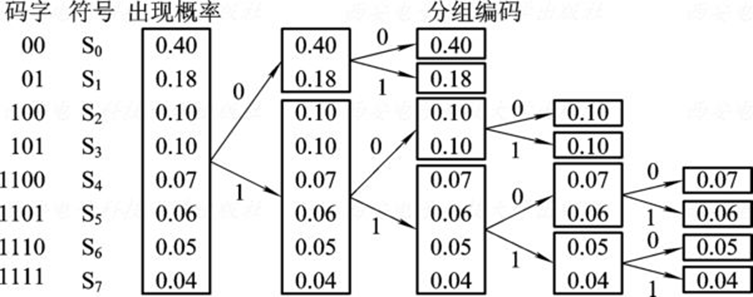### 9.4 算术编码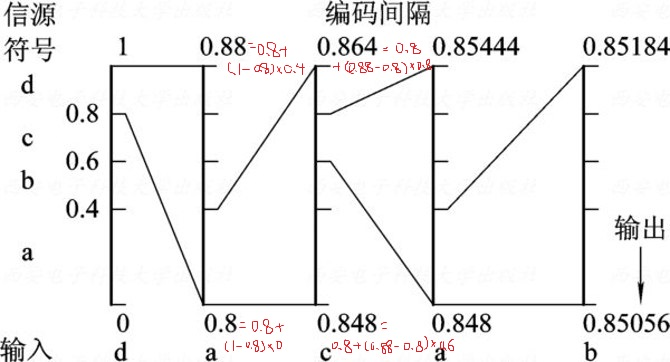### 9.5 行程编码

aabbbcddddd=2a3b1c5d

### 9.6 LZW编码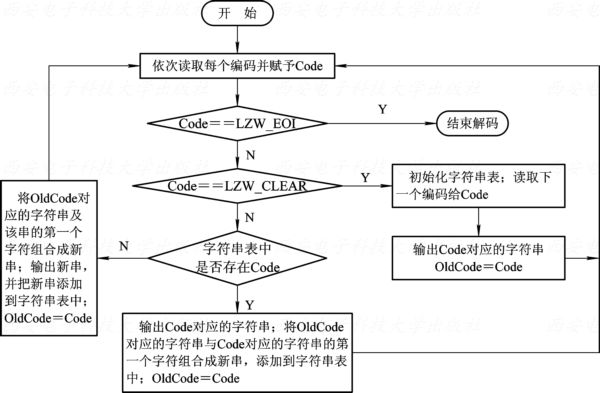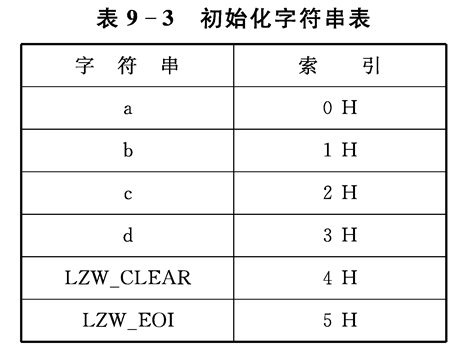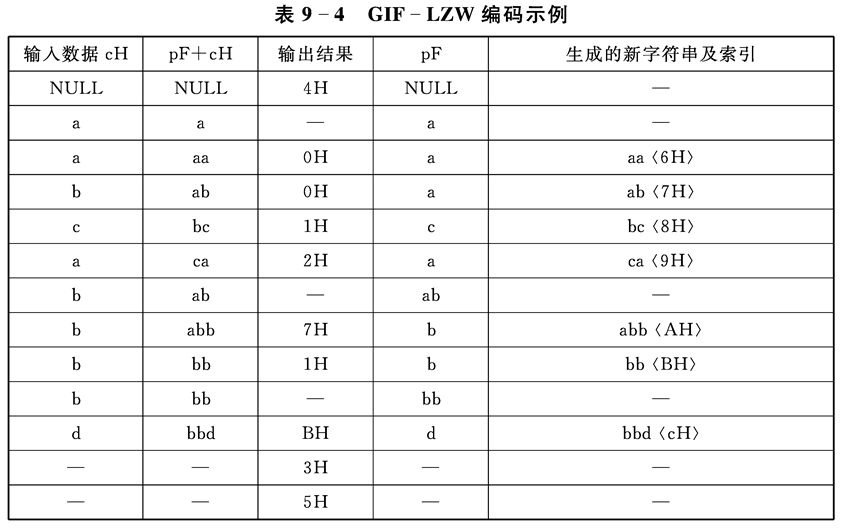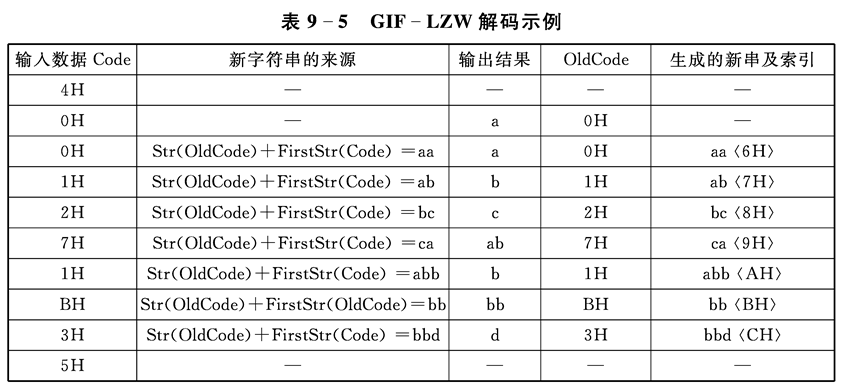## 数字图像处理（第三版）——图像处理方法

2018-11-27 23:27:12 Aidam_Bo 阅读数 866
• ###### 目标跟踪

学会自己查阅opencv doxygen文档 学会编写opencv代码实现简单图像处理变换 初步了解图像算法及机器视觉基础 运用计算机视觉相关知识和opencv库来构建简单的应用程序。

231人学习 金圣韬
免费试看

在实际的图像分割中，往往只用到一阶和二阶导数，虽然，原理上，可以用更高阶的导数，但是，因为噪声的影响，在纯粹二阶的导数操作中就会出现对噪声的敏感现象，三阶以上的导数信息往往失去了应用价值。二阶导数还可以说明灰度突变的类型。在有些情况下，如灰度变化均匀的图像，只利用一阶导数可能找不到边界，此时二阶导数就能提供很有用的信息。二阶导数对噪声也比较敏感，解决的方法是先对图像进行平滑滤波，消除部分噪声，再进行边缘检测。不过，利用二阶导数信息的算法是基于过零检测的，因此得到的边缘点数比较少，有利于后继的处理和识别工作。

各种算子的存在就是对这种导数分割原理进行的实例化计算，是为了在计算过程中直接使用的一种计算单位。

1.Sobel算子

其主要用于边缘检测，在技术上它是以离散型的差分算子，用来运算图像亮度函数的梯度的近似值， Sobel算子是典型的基于一阶导数的边缘检测算子，由于该算子中引入了类似局部平均的运算，因此对噪声具有平滑作用，能很好的消除噪声的影响。Sobel算子对于象素的位置的影响做了加权，与Prewitt算子、Roberts算子相比因此效果更好。

Sobel算子包含两组3x3的矩阵，分别为横向及纵向模板，将之与图像作平面卷积，即可分别得出横向及纵向的亮度差分近似值。实际使用中，常用如下两个模板来检测图像边缘。

2. Isotropic Sobel算子

Sobel算子另一种形式是(Isotropic Sobel)算子，加权平均算子，权值反比于邻点与中心点的距离，当沿不同方向检测边缘时梯度幅度一致，就是通常所说的各向同性Sobel(Isotropic Sobel)算子。模板也有两个，一个是检测水平边沿的 ，另一个是检测垂直平边沿的 。各向同性Sobel算子和普通Sobel算子相比，它的位置加权系数更为准确，在检测不同方向的边沿时梯度的幅度一致。

3. Roberts算子

Roberts边缘算子是一个2x2的模板，采用的是对角方向相邻的两个像素之差。从图像处理的实际效果来看，边缘定位较准，对噪声敏感。适用于边缘明显且噪声较少的图像分割。Roberts边缘检测算子是一种利用局部差分算子寻找边缘的算子,Robert算子图像处理后结果边缘不是很平滑。经分析，由于Robert算子通常会在图像边缘附近的区域内产生较宽的响应，故采用上述算子检测的边缘图像常需做细化处理，边缘定位的精度不是很高。

4. Prewitt算子

Prewitt算子是一种一阶微分算子的边缘检测，利用像素点上下、左右邻点的灰度差，在边缘处达到极值检测边缘，去掉部分伪边缘，对噪声具有平滑作用 。其原理是在图像空间利用两个方向模板与图像进行邻域卷积来完成的，这两个方向模板一个检测水平边缘，一个检测垂直边缘。

对数字图像f(x，y)，Prewitt算子的定义如下：

G(i)=|[f(i-1,j-1)+f(i-1,j)+f(i-1，j+1)]-[f(i+1,j-1)+f(i+1，j)+f(i+1，j+1)]|

G(j)=|[f(i-1,j+1)+f(i,j+1)+f(i+1，j+1)]-[f(i-1,j-1)+f(i,j-1)+f(i+1，j-1)]|

Prewitt算子对噪声有抑制作用，抑制噪声的原理是通过像素平均，但是像素平均相当于对图像的低通滤波，所以Prewitt算子对边缘的定位不如Roberts算子。

5.Laplacian算子

Laplace算子是一种各向同性算子，二阶微分算子，在只关心边缘的位置而不考虑其周围的象素灰度差值时比较合适。Laplace算子对孤立象素的响应要比对边缘或线的响应要更强烈，因此只适用于无噪声图象。存在噪声情况下，使用Laplacian算子检测边缘之前需要先进行低通滤波。所以，通常的分割算法都是把Laplacian算子和平滑算子结合起来生成一个新的模板。

拉式算子用来改善因扩散效应的模糊特别有效，因为它符合降制模型。扩散效应是成像过程中经常发生的现象。

Laplacian算子一般不以其原始形式用于边缘检测，因为其作为一个二阶导数，Laplacian算子对噪声具有无法接受的敏感性；同时其幅值产生算边缘，这是复杂的分割不希望有的结果；最后Laplacian算子不能检测边缘的方向；所以Laplacian在分割中所起的作用包括：（1）利用它的零交叉性质进行边缘定位；（2）确定一个像素是在一条边缘暗的一面还是亮的一面；一般使用的是高斯型拉普拉斯算子（Laplacian of a Gaussian,LoG)，由于二阶导数是线性运算，利用LoG卷积一幅图像与首先使用高斯型平滑函数卷积改图像，然后计算所得结果的拉普拉斯是一样的。所以在LoG公式中使用高斯函数的目的就是对图像进行平滑处理，使用Laplacian算子的目的是提供一幅用零交叉确定边缘位置的图像；图像的平滑处理减少了噪声的影响并且它的主要作用还是抵消由Laplacian算子的二阶导数引起的逐渐增加的噪声影响。

6.Canny算子

该算子功能比前面几种都要好，但是它实现起来较为麻烦，Canny算子是一个具有滤波，增强，检测的多阶段的优化算子，在进行处理前，Canny算子先利用高斯平滑滤波器来平滑图像以除去噪声，Canny分割算法采用一阶偏导的有限差分来计算梯度幅值和方向，在处理过程中，Canny算子还将经过一个非极大值抑制的过程，最后Canny算子还采用两个阈值来连接边缘。

Canny边缘检测算法

step1: 用高斯滤波器平滑图象；

step2: 用一阶偏导的有限差分来计算梯度的幅值和方向；

step3: 对梯度幅值进行非极大值抑制

step4: 用双阈值算法检测和连接边缘

(1)图象边缘检测必须满足两个条件：一能有效地抑制噪声；二必须尽量精确确定边缘的位置。

(2)根据对信噪比与定位乘积进行测度，得到最优化逼近算子。这就是Canny边缘检测算子。

(3)类似与Marr（LoG）边缘检测方法，也属于先平滑后求导数的方法。

## 【OpenCV学习笔记】之五 RGB图像归一化处理函数，消除线性变化的光照影响

2013-06-19 20:02:34 zfdxx369 阅读数 7642
• ###### 目标跟踪

学会自己查阅opencv doxygen文档 学会编写opencv代码实现简单图像处理变换 初步了解图像算法及机器视觉基础 运用计算机视觉相关知识和opencv库来构建简单的应用程序。

231人学习 金圣韬
免费试看

听说，将RGB图像归一化之后，可以消除部分光照影响，于是在没有找着现成的之后试着写了个，发现它并不能解决我的问题， 处理之后的视觉效果比较差还，像素之间显得那么不和谐。// 将BGR颜色归一化，消除线性光照影响
bool colorNormal(Mat& img)
{
if (img.channels() != 3)
{
return false;
}

int nl= img.rows; // number of lines
int nc= img.cols ; // number of columns
// is it a continous image?
if (img.isContinuous())
{
nc= nc*nl;
nl= 1;  // it is now a 1D array
}

double bgrSum;
// for all pixels
for (int j=0; j<nl; j++)
{
// pointer to first column of line j
uchar* data= img.ptr(j);
for (int i=0; i<nc; i++)
{
// process each pixel --------
bgrSum= 255.0/(1 +data +data +data);
data *=bgrSum;
data *=bgrSum;
data *=bgrSum;
data +=3;
}
}

return true;

}